## A 1.0-kg cart and a 0.50-kg cart sit at different positions on a low-friction track. You push on the 1.0-kg cart with a constant 4.0-N force

Question

A 1.0-kg cart and a 0.50-kg cart sit at different positions on a low-friction track. You push on the 1.0-kg cart with a constant 4.0-N force for 0.20 m. You then remove your hand, and the cart slides 0.35 m and strikes the 0.50-kg cart. What is the work done by you on the two-cart system? How far does the system’s center of mass move while you are pushing the 1.0-kg cart? By what amount does your force change the kinetic energy of the system’s center of mass?

in progress 0
1 month 2021-08-04T09:21:19+00:00 1 Answers 3 views 0

a)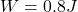b)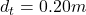c)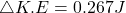Explanation:

From the question we are told that:

Mass of cart 1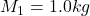Mass of cart 1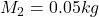Force on  cart 1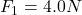Push Distance of cart 1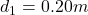Slide Distance of cart 1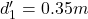a)

Generally the equation for work-done is mathematically given by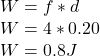b)

The systems center of mass moved a net totally of (while being pushed)

Mass 1 =0.20m

Mass 2=0

Therefore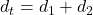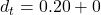c)

Since work-done is equal to K.E energy of cart 1

Therefore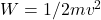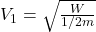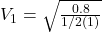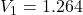Therefore Kinetic energy before collision is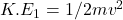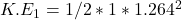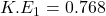Generally from the equation for conservation  of momentum the Velocity of cart 2 is mathematically given by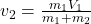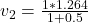Therefore the final K.E is mathematically given by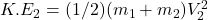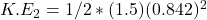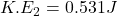Generally the Change in K.E is mathematically given by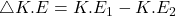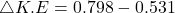Therefore the will force change the kinetic energy of the system’s center of mass by